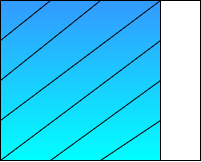What fraction is represented by the given figure?A. $\dfrac{1}{3}$B. $\dfrac{2}{4}$C. $\dfrac{1}{2}$D. We cannot sayVerified
147.6k+ views
Hint: In this question remember to observe the given figure and differentiate the shaded and unshaded parts and also remember that the shaded and unshaded parts are not defined, this information will help you to approach the solution of the question.Note: In the above solution we came across the term “fraction” which can be explained as the representation of the part of a whole object or part this can be explained with the help of an example. Suppose we have an apple and we sliced it into 6 pieces so the representation of 2 pieces of apple will be given by; the fraction of 2 pieces of apple = number of required apple pieces/total numbers of apple pieces so the fraction of 2 pieces of apple will be $\dfrac{2}{6} = \dfrac{1}{3}$.